# OECD Threatened species data analysis 3 - ANOVA(ANalysis Of VAriance) without lm() and anova()

In this brlog, let's do ANOVA(Analysis of Variance).

We see average Value(percentage of threatened species) are different by SUBJECT.BIRD has the highest Value and PLANT has the lowest.
But this difference is statistically significant?

Let's check it in R. We can use lm() function.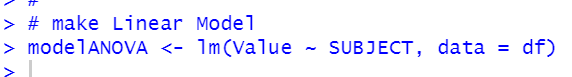Use anova() to see the results.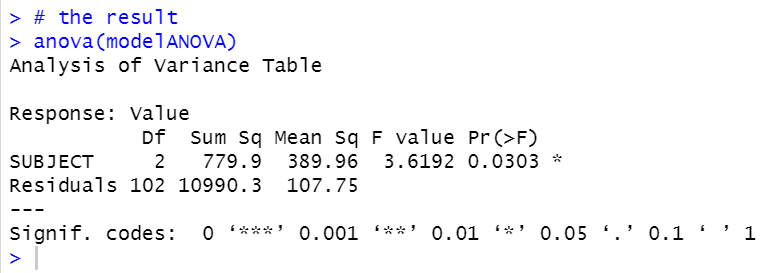p-value is 0.0303, so it is significant at 5% significant level.

Now, let's do ANOVA without lm() and summary() function.

1. calculate overall average.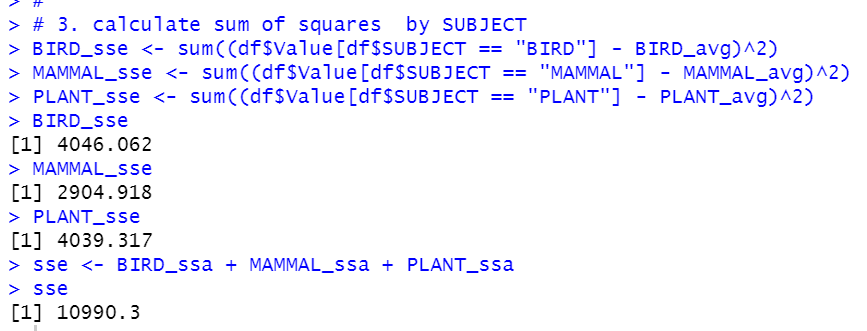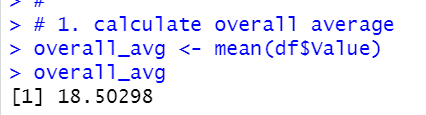2. calculate average by SUBJECT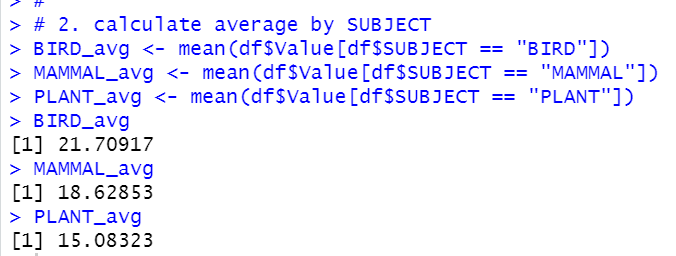3. calculate variance by SUBJECTWe get 10990.3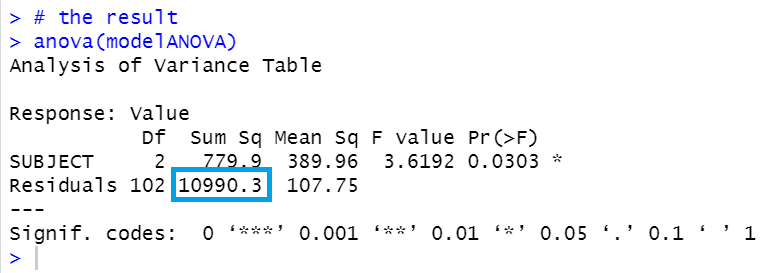4. Calculate overall sum of squares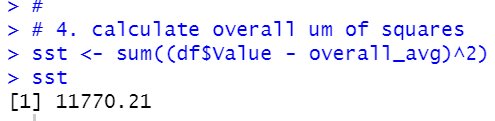5. Calculate SSE = SST - SSA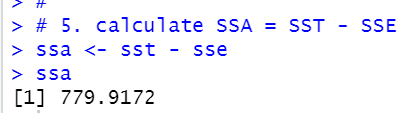We got 779.9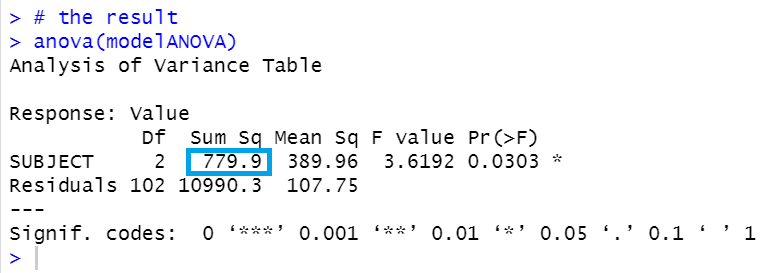6. calculate degree of freedom for SUBJECT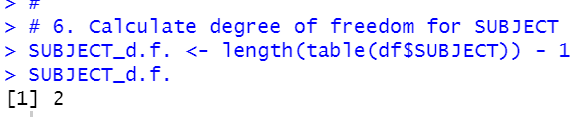We get 2.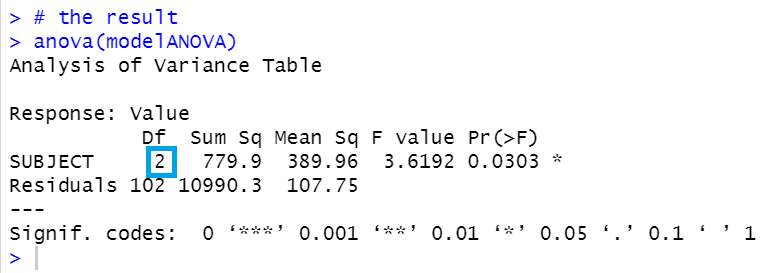7. Calculatedegree of freedom for Residuals.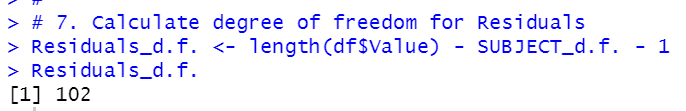We got 102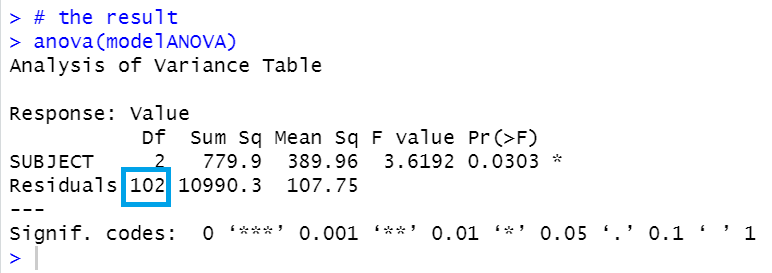Now, we got 2, 102, 779.9 and 10990.3.

8. we can calculate Mean Sq.

SUBJECT Mean Sq = 779.9 / 2 = 389.96

Residuals Mean Sq = 10990.3 / 102 = 107.75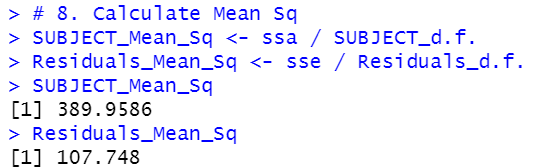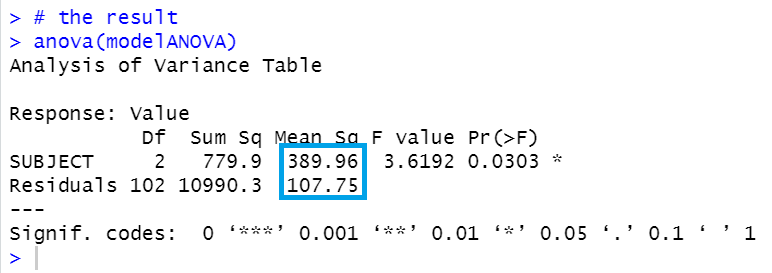9. Calculate F-value.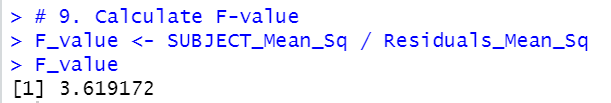We got 3.6192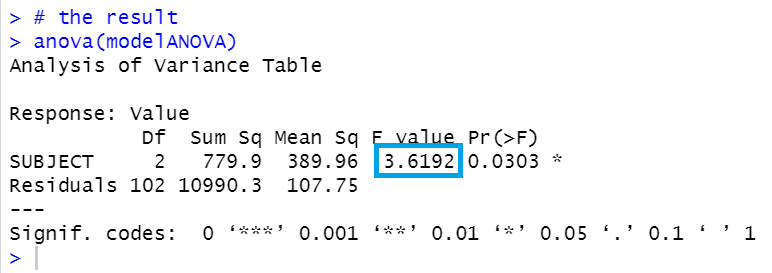10. Calculate p-value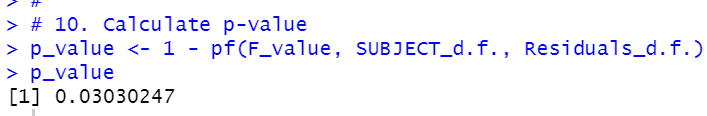Nice! Finally we got 0.0303!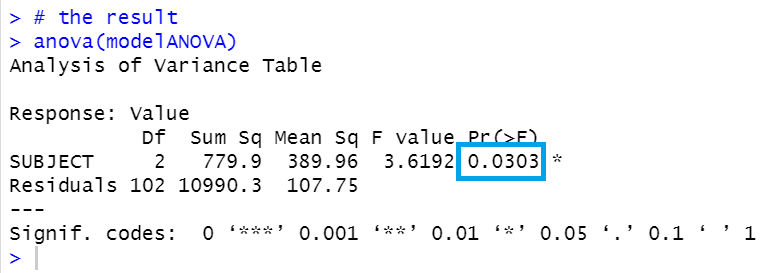That's it.

Next blog is...

If you would like to see the first blog.